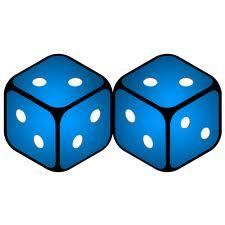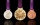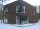# Event probability

The probability of event P in 8 independent experiments is 0.33. What is the probability that the event P occurs in one experiment (probability is same)?

Result

p =  87.1 %

#### Solution:

$p = 100 \sqrt[ 8]{ 0.33} = 87.1 \%$Our examples were largely sent or created by pupils and students themselves. Therefore, we would be pleased if you could send us any errors you found, spelling mistakes, or rephasing the example. Thank you!

Leave us a comment of this math problem and its solution (i.e. if it is still somewhat unclear...):Be the first to comment!Tips to related online calculators
Would you like to compute count of combinations?

## Next similar math problems:

1. Auto-tuningDuring auto-tuning, the TV searched for 25 channels, four of which were music. The channels are stored in the TV's memory in random order. Express the percentage of the event that the music channel will be saved first.
2. RewardJanko and Peter divided the reward from the brigade so that Peter got 5/8 of the reward. What percentage of Janko's reward got?
3. ResearchersResearchers ask 200 families whether or not they were the homeowner and how many cars they had. Their response was homeowner: 14 no car or one car, two or more cars 86, not homeowner: 38 no car or one car, two or more cars 62. What percent of the families
4. Theorem proveWe want to prove the sentence: If the natural number n is divisible by six, then n is divisible by three. From what assumption we started?
5. Percentages52 is what percent of 93?
6. The Chemistry testThe Chemistry test contained 8 questions, each with 3 points. Peter scored 21 points. How many percent did Peter write a test?
7. Percentage increaseIncrease number 400 by 3.5%
8. SummerjobThe temporary workers planted new trees. Of the total number of 500 seedlings, they managed to plant 426. How many percents did they meet the daily planting limit?
9. PercentsHow many percents is 900 greater than the number 750?
10. PowerNumber ?. Find the value of x.
11. CalculationHow much is sum of square root of six and the square root of 225?Calculate how many percent will increase the length of an HTML document, if any ASCII character unnecessarily encoded as hexadecimal HTML entity composed of six characters (ampersand, grid #, x, two hex digits and the semicolon). Ie. space as: &#x20;The ball was discounted by 10 percent and then again by 30 percent. How many percent of the original price is now?Persons surveyed:100 with result: Volleyball=15% Baseball=9% Sepak Takraw=8% Pingpong=8% Basketball=60% Find the average how many like Basketball and Volleyball. Please show your solution.One half of ? is: ?Express the expression ? as the n-th power of the base 10.The highway repair was planned for 15 days. However, it was reduced by 30%. How many days did the repair of the highway last?